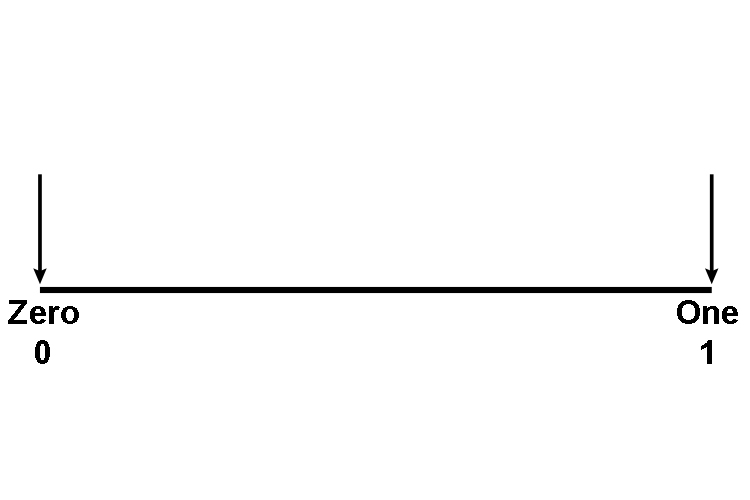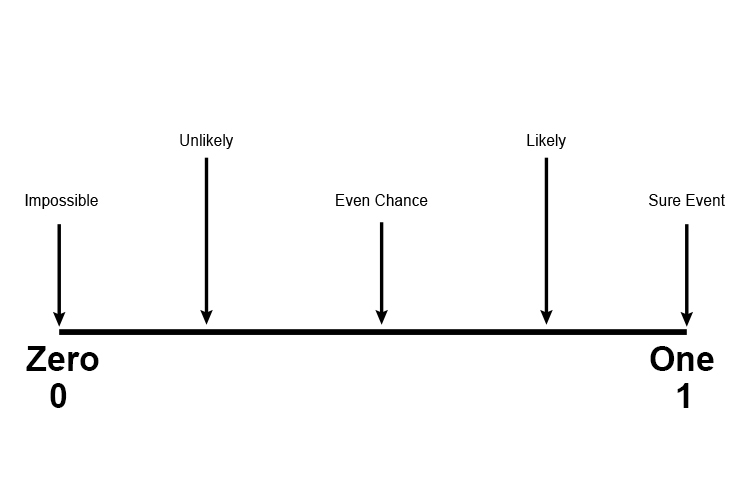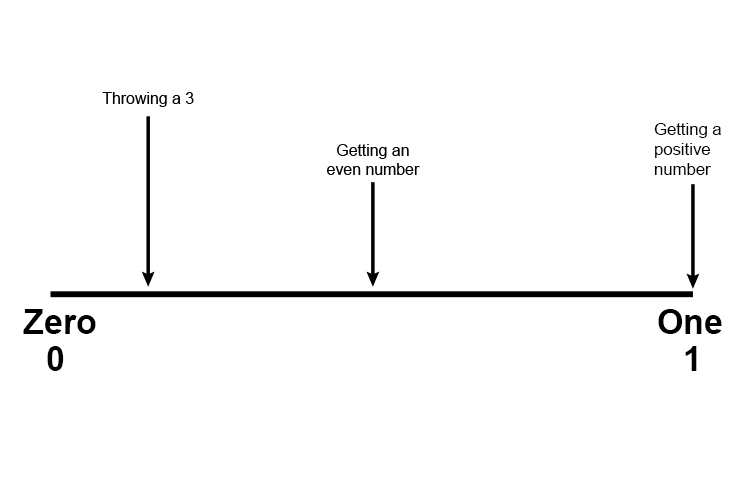# Probability Line Examples

Example 1

Put these words in order on a probability line:

Sure event, Unlikely, Likely, Impossible and even chance.

1.  Draw a line with the probability at each end2.  Put the words in order:

Impossible, Unlikely, Even chance, Likely, Sure event

3.  Place these on the probability line:Example 2

Represent the given events on a probability line.

a) Getting a 3 in a throw of a dice

b) Getting an even number in a throw of a dice

c) Getting a positive number in a throw of a dice

1.  Draw a line with the probability at each end2.  Work out the probability for each of a), b), and c) occurring.

a) Getting a 3 in a throw of a dice =1/6=0.167

b) Getting an even number in a throw of a dice =3/6=1/2=0.5

c) Getting a positive number in a throw of a dice =6/6=1

3.  Place these on the probability line: